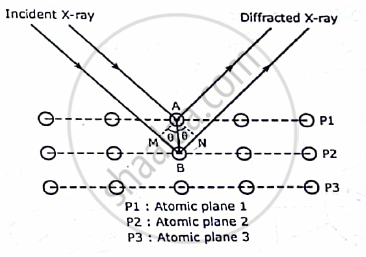# Explain with Example How to Determine Crystal Structure by Bragg’S X-ray Spectrometer. - Applied Physics 1

Short Note

Explain with example how to determine crystal structure by Bragg’s X-ray spectrometer.

#### Solution

W.L Bragg’s explained the phenomenon of X-ray diffraction from a single crystal shown as follows
When a beam of X-rays is incident on a crystal it is scattered by individual atoms of the rich atomic planes. Thus, each atom become a source of scattered radiation. The atomic planes responsible for the X-ray diffraction are called BRAGG’S PLANES. Therefore the sets of Braggs planes constitute the crystal grating. Bragg’s scattering or Bragg’s diffraction is also referred as Braggs reflection. Bragg derived a law called Bragg’s law to explain the X-ray diffraction effect.
Here a beam of X-ray is incident on a set of parallel planes of a crystal. The rays makes a glancing angle θ and are practically reflected from different successive planes. The phase relationship of the scattered rays can be determined from their path differences. Here two parallel X-rays are  reflected from two consecutive planes PI and P2. The path differences between then as shownδ = MB + BN = 2MB = 2AB sinθ
Here AB = d, the interplanar spacing of the crystal. Hence,
δ = 2dsinθ
The two diffracted rays reinforce each other when they interfere constructively when their path difference δ is equal to nλ
Hence,              2dsinθ = n λ
This is called Bragg’s law.

Concept: Bragg’S Law
Is there an error in this question or solution?